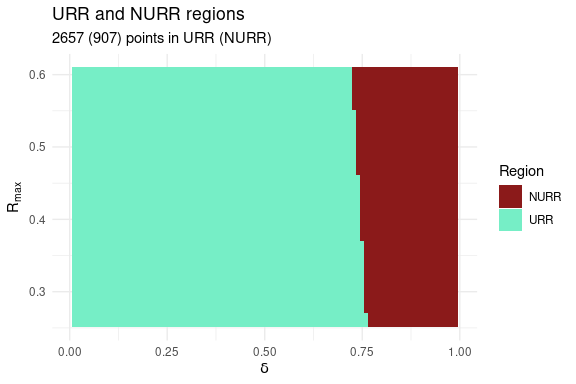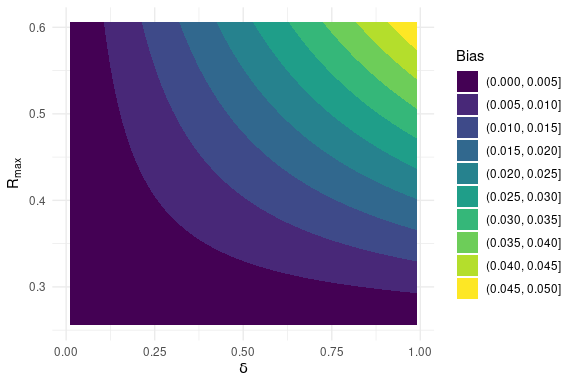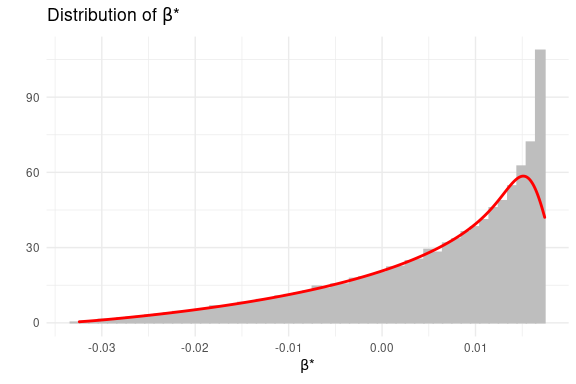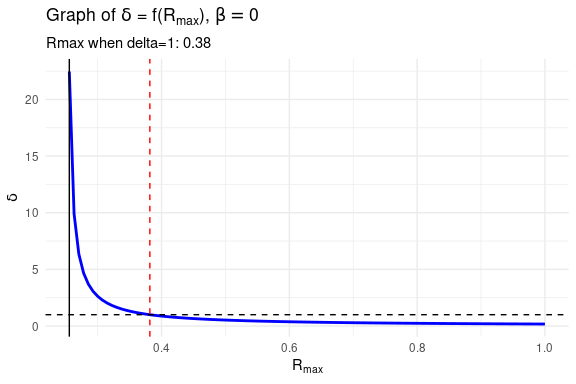# Introduction

## Four Regressions

The goal of the bate package is to present some functions to compute quantiles of the empirical distribution of the bias-adjusted treatment effect (BATE) in a linear econometric model with omitted variables. To analyze such models, a researcher should consider four regression models: (a) a short regression model where the outcome variable is regressed on the treatment variable, with or without additional controls; (b) an intermediate regression model where additional control variables are added to the short regression; (c) a hypothetical long regression model, where an index of the omitted variable(s) is added to the intermediate regressions; and (d) an auxiliary regression where the treatment variable is regressed on all observed (and included) control variables.

As an example, suppose a researcher has estimated the following model, $y = \alpha + \beta_1 x + \gamma_1 w_1 + \gamma_2 w_2 + \varepsilon,$ and is interested in understanding the impact of some omitted variables on the results. In this case:

• outcome variable: $$y$$
• treatment variable: $$x$$
• short regression: $$y$$ regressed on $$x$$;
• intermediate regression: $$y$$ regressed on $$x, w_1, w_2$$;
• auxiliary regression: $$x$$ regressed on $$w_1, w_2$$;
• hypothetical long regression: $$y$$ regressed on $$x, w_1,w_2$$ and the omitted variables;

In this example, the estimated treatment effect is $$\hat{\beta}_1$$. In the presence of omitted variables, $$\hat{\beta}_1$$ is a biased estimate of the true treatment effect, $$\beta_1$$. The functions in this package will allow researchers to create quantiles of the empirical distribution of the BATE, i.e. the treatment effect once we have adjusted for the effect of omitted variable bias. We will denote the BATE as $$\beta^*$$.

The researcher will need to supply the data set (as a data frame), the name of the outcome variable, the name of the treatment variable, and the names of the additional regressors in the intermediate regression. The functions in this package will then compute the quantiles of the empirical distribution of BATE, $$\beta^*$$.

## Two Important Parameters

Two parameters capture the effect of the omitted variables in this set up.

The first parameter is $$\delta$$. This captures the relative strength of the unobservables, compared to the observable controls, in explaining variation in the treatement variable. In the functions below this is denoted as the parameter delta. This parameter is a real number and can take any value on the real line, i.e. it is unbounded. Hence, in any specific analysis, the researcher will have to choose a lower and an upper bound for delta. For instance, if in any empirical analysis, the researcher believes, based on knowledge of the specific problem being investigated, that the unobservables are less important than the observed controls in explaining the variation in the treatment variable, then she could choose delta to lie between 0 and 1. On the other hand, if she believes that the unobservables are more important than the observed controls in explaining the variation in the treatment variable, then she should choose delta to lie between 1 and 2 or 1 and 5.

The second parameter is $$R_{max}$$. This captures the relative strength of the unobservables, compared to the observable controls, in explaining variation in the outcome variable. In the functions below, this is captured by the parameter Rmax. The parameter Rmax is the R-squared in the hypothetical long regression. Hence, it lies between the R-squared in the intermediate regression ($$\tilde{R}$$) and 1. Since the lower bound of Rmax is given by $$\tilde{R}$$, in any specific analysis, the researcher will only have to choose an upper bound for Rmax.

In a specific empirical analysis, a researcher will use domain knowledge about the specific issue under investigation to determine a plausible range for delta (e.g. $$0.01 \leq \delta \leq 0.99$$). This will be given by the interval on the real line lying between deltalow and deltahigh (the researcher will choose deltalow and deltahigh). Using the example in this paragraph, deltalow=0.01 and deltahigh=0.99.

In a similar manner, a researcher will use domain knowledge about the specific issue under investigation to determine Rmax. Here, it will be important to keep in mind that Rmax is the R-squared in the hypothetical long regression. Now, it is unlikely that including all omitted variables and thereby estimating the hypothetical long regression will give an R-squared of 1. This is because, even after all the regressors have been included, some variation of the outcome might be plausibly explained by a stochastic element. Hence, Rmax will most likely be different from, and less than, 1. This will be denoted by Rhigh (e.g. Rmax=0.61).

## The Algorithm

How is the omitted variable bias and the BATE computed? The key result that is used to compute the BATE is this: the omitted variable bias is the real root of the following cubic equation $a \nu^3 + b \nu^2 + c \nu + d =0,$ where

• $$a = (\delta -1)(\tau_X \sigma_X^2 - \tau_X^2) \neq 0,$$
• $$b = \tau_X \left( \mathring{\beta} - \tilde{\beta}\right) \sigma^2_X \left( \delta - 2 \right),$$
• $$c = \delta \left( R_{max} - \tilde{R} \right) \sigma^2_Y \left( \sigma^2_X - \tau_X \right) - \left( \tilde{R} - \mathring{R} \right) \sigma^2_Y \tau_X - \sigma^2_X \tau_X \left( \mathring{\beta} - \tilde{\beta}\right)^2,$$ and
• $$d = \delta \left( R_{max} - \tilde{R} \right) \sigma^2_Y \left( \mathring{\beta} - \tilde{\beta}\right) \sigma^2_X,$$

where, in turn,

• $$\sigma_Y^2$$ is the variance of the outcome variable,
• $$\sigma_X^2$$ is the variance of the treatment variable,
• $$\mathring{\beta}$$ is the treatment effect in the short regression,
• $$\mathring{R}$$ is the R-squared in the short regression,
• $$\tilde{\beta}$$ is the treatment effect in the intermediate regression,
• $$\tilde{R}$$ is the R-squared in the intermediate regression, and
• $$\tau_X^2$$ is the variance of the residual in the auxiliary regression.

Hence, we see that the coefficients of the cubic equation are functions of the variances of the outcome and treatment variables ($$\sigma_Y^2, \sigma_X^2$$), parameters of the short regression ($$\mathring{\beta}, \mathring{R}$$), intermediate regression ($$\tilde{\beta}, \tilde{R}$$) and auxiliary regression ($$\tau_X^2$$), and the values of delta and Rmax. The important result is that the omitted variable bias is the real root of the above cubic equation (Proposition 2, Oster, 2019; Proposition 1 and 2 in Basu, 2022).

In a specific empirical analysis, the variances of the outcome and treatment variables, and the parameters of the short, intermediate and auxiliary regressions are known. Hence, the coefficients of the cubic equation become functions of delta and Rmax, the two key parameters that the researcher chooses, using domain knowledge.

Once the researcher has chosen deltalow, deltahigh and Rhigh, this defines a bounded box on the (delta, Rmax) plane defined by the Cartesian product of the interval [deltalow, deltahigh] and of the interval [Rlow, Rhigh]. The main functions in this package computes the root of the cubic equation on a sufficiently granular grid (the degree of granularity will be chosen by the user) covering the bounded box.

To compute the root of the cubic equation, the algorithm first evaluates the discriminant of the cubic equation on each point of the grid and partitions the box into two regions: (a) unique real root (URR) and NURR (no unique real root). There are three cases to consider.

• Case 1: If all points of the bounded box are in URR, then the algorithm chooses the unique real root of the cubic at each point as the estimate of the omitted variable bias.
• Case 2: If some non-empty part of the box is in NURR, then the algorithm first computes roots on the URR region, and then, starting from the boundary points of URR/NURR, covers points on the NURR in small steps. At each step, the algorithm chooses the real root at a grid point in the NURR that is closest in absolute value to the real root at a previously selected grid point. Continuity of the roots of a polynomial with respect to its coefficients guarantees that the algorithm selects the correct real root at each point.
• Case 3: If the bounded box is completely contained in NURR, then the algorithm extends the size of the box in small steps in the delta direction to generate a nonempty intersection with a URR region. Once that is found, the algorithm implements the steps outlined in step 2.

The bias is then used to compute the BATE, $$\beta^*$$, which is defined as the estimated treatment effect in the intermediate regression minus the bias, i.e. $\beta^* = \tilde{\beta}-\nu,$ where $$\beta^*$$ is the bias-adjusted treatment effect, $$\tilde{\beta}$$ is the treatment effect estimated from the intermediate regression and $$\nu$$ is the real root of the relevant cubic equation.

The functions in this package will compute $$\beta^*$$ at each point of the grid that covers the bounded box chosen by the researcher. Hence, this will generate a large vector of values of $$\beta^*$$ and we can use this to compute the empirical distribution of $$\beta^*$$, the BATE. Asymptotic theory shows that the BATE converges in probability to the true treatment effect, i.e. $\beta^* \overset{p}{\to} \beta.$ Hence, the interval defined by the 2.5-th and 97.5-th quantiles of the empirical distribution of the BATE will contain the true treatment effect with 95 percent probability.

## The Main Functions

This package provides three functions to compute quantiles of the empirical distribution of the omitted bias and BATE, ovbias(), ovbias_lm() and ovbias_par(). These functions implement the same algorithm to compute the empirical distribution of the bias and BATE and only differ in how the user provides the parameters of the short, intermediate and auxiliary regressions. But before we discuss the main functions, let us look at two other functions that are useful.

An useful function to collect relevant parameters from the short, intermediate and auxiliary regressions is:

• collect_par(): collects parameters from the short, intermediate and auxiliary regressions; (user provides name of the data set, name of outcome variable, name of treatment variable, names of control variables in the short regression, if relevant, and names of additional variables in the intermediate regression); the output of this function is a data frame.

Users can use the output from collect_par() to construct an area plot of the bounded box using:

• urrplot(): creates a colored area plot of the bounded box chosen by the user demarcating the area where the cubic equation has unique real root (URR) from the area where the cubic equation has three real roots (NURR); the output is a plot object.

### ovbias() function

To use the ovbias() function, the user will need to run first collect the parameters from the short, intermediate and auxiliary regressions, using the collect_par() function and then feed it into the ovbias() function, along with the following:

• deltalow: lower limit of delta (e.g. 0.01)
• deltahigh: upper limit of delta (e.g. 0.99)
• Rhigh: upper limit of Rmax (e.g. 0.61)
• e: step size in defining the grid (e.g. 0.01)

In using the collect_par() function, the user will need to specify the following:

• data: the name of the data frame (e.g. NLSY_IQ)
• outcome: name of the outcome variable in double quotes (e.g. “iq_std”)
• treatment: name of the treatment variable in double quotes (e.g. “BF_months”)
• control: names of additional regressors to include in the intermediate regression, supplied as a vector (e.g. c(“age”,“sex”,“income”,“motherAge”,“motherEDU”,“mom_married”,“race”))
• other_regressors: names of regressors in the short regression, other than the treatment variable, supplied as a vector (e.g. c(“sex”,“age”))

The output of the ovbias() function is a list of three elements.

• Data: A data frame containing the bias (bias) and bias-adjusted treatment effect (bstar) for each point on the grid.
• bias_Distribution: Quantiles (2.5,5.0,50,95,97.5) of the empirical distribution of bias.
• bstar_Distribution: Quantiles (2.5,5.0,50,95,97.5) of the empirical distribution of the BATE (bstar).

### ovbias_par() function

To use the ovbias_par() function, the user needs to specify the following:

• data: the name of the data frame (e.g. NLSY_IQ)
• outcome: name of the outcome variable in double quotes (e.g. “iq_std”)
• treatment: name of the treatment variable in double quotes (e.g. “BF_months”)
• control: names of additional regressors to include in the intermediate regression, supplied as a vector (e.g. c(“age”,“sex”,“income”,“motherAge”,“motherEDU”,“mom_married”,“race”))
• other_regressors: names of regressors in the short regression, other than the treatment variable, supplied as a vector (e.g. c(“sex”,“age”))
• deltalow: lower limit of delta (e.g. 0.01)
• deltahigh: upper limit of delta (e.g. 0.99)
• Rhigh: upper limit of Rmax (e.g. 0.61)
• e: step size in defining the grid (e.g. 0.01)

The output of the ovbias_par() function is identically same with the output of the ovbias() function.

### ovbias_lm() function

To use the ovbias_lm() function, the user needs to specify three lm objects that capture the short, intermediate and auxiliary regressions:

• lm_shrt: lm object for the short regression
• lm_int: lm object for the intermediate regression
• lm_aux: lm object for the auxiliary regression
• deltalow: lower limit of delta (e.g. 0.01)
• deltahigh: upper limit of delta (e.g. 0.99)
• Rhigh: upper limit of Rmax (e.g. 0.61)
• e: step size in defining the grid (e.g. 0.01)

The output of the ovbias_lm() function is identically same with the output of the ovbias() function.

Using the output from ovbias(), ovbias_par() or ovbias_lm(), users can construct various plots to visualize the results:

• cplotbias(): contour plot of the bias over the bounded box; the output of this function is a plot object;
• dplotbate(): histogram and density plot of BATE; the output of this function is a plot object;

The methodology proposed in Basu (2022) is slightly different from, and a critique of, Oster (2019). Hence, it might be useful to compare the results of the two methodologies. The methodology proposed in Oster (2019) is implemented via these functions:

• osterbds(): identified sets according to Oster’s methodology; the output of this function is a data frame;
• osterdelstar(): the value of $$\delta^*$$ for a chosen value of $$R_{max}$$; the output of this function is a data frame;
• delfplot(): a plot of the graph of the function, $$\delta=f(R_{max})$$; the output of this function is a plot object.

## Example: Impact of Maternal Behavior on Child IQ

Install the package from CRAN and then load it.

library(bate)

### Setting Up

Let us load the data set.

data("NLSY_IQ")

The data set has two .RData objects: NLSY_IQ (to be used for the analysis of maternal behavior on child IQ) and NLSY_BW (to be used for the analysis of maternal behavior on child birthweight).

In this example, we will analyse the effect of maternal behavior on child IQ scores. Let us start out by seeing the names of the variables in the NLSY_IQ data set.

names(NLSY_IQ)
#>   "iq_std"             "BF_months"          "mom_drink_preg_all"
#>   "lbw_preterm"        "age"                "female"
#>   "black"              "motherAge"          "motherEDU"
#>  "mom_married"        "income"             "sex"
#>  "race"

For use in the example below, let us set age and race as factor variables

NLSY_IQ$age <- factor(NLSY_IQ$age)
NLSY_IQ$race <- factor(NLSY_IQ$race)

Let us use the vtable package to see the summary statistics of the variables in our data set.

library(vtable)
vtable::st(NLSY_IQ)
Summary Statistics
Variable N Mean Std. Dev. Min Pctl. 25 Pctl. 75 Max
iq_std 6514 0.017 0.99 -4.136 -0.586 0.693 3.467
BF_months 6514 2.403 4.63 0 0 3 48
mom_drink_preg_all 6090 0.323 0.468 0 0 1 1
lbw_preterm 5837 0.051 0.219 0 0 0 1
age 6514
… 4 1794 27.5%
… 5 1847 28.4%
… 6 1075 16.5%
… 7 922 14.2%
… 8 876 13.4%
female 6514 0.491 0.5 0 0 1 1
black 6514 0.282 0.45 0 0 1 1
motherAge 6514 25.201 5.704 14 21 28 45
motherEDU 6514 12.189 2.577 1 11 13 95
mom_married 6514 0.652 0.476 0 0 1 1
income 6514 40799.978 80453.373 0 11474 45000 974100
sex 6514 1.491 0.5 1 1 2 2
race 6514
… 1 1328 20.4%
… 2 1838 28.2%
… 3 3348 51.4%

### Setting Up the Analysis

We will work with an example where the effect of months of breastfeeding on children’s IQ score is analyzed. Thus, here, the outcome variable is a child’s IQ score and the treatment variable is the months of breastfeeding by the mother. Additional control variables are: sex and age of the child, and the mother’s age, the mother’s years of formal education, whether the mother is married and the race of the mother. For further details of this example, see section 4.2 in Oster (2019). For ease of reference, let us note that we are working with the model reported in the first row of Table 3 in Oster (2019) and the first block of 4 rows in Table 2 in Basu (2022).

Using the names of variables in the data set, we have: * short regression: iq_std ~ BF_months + sex + age * intermediate regression: iq_std ~ BF_months + sex + age + income + motherAge + motherEDU + mom_married + race.

Let us use the collect_par() function to collect parameters from the short, intermediate and auxiliary regressions. It is important to note that other_parameters option in this function should refer to a subset of control. If the researcher fails to ensure this, the collect_par() function will throw an error.

parameters <- bate::collect_par(data=NLSY_IQ,
outcome="iq_std",
treatment="BF_months",
control=c("age","sex","income","motherAge","motherEDU","mom_married","race"),
other_regressors = c("sex","age"))

Let us see the parameters by looking at the object parameters that we used to store the output of the collect_par() function.

(parameters)
#>                beta0         R0  betatilde   Rtilde    sigmay   sigmax     taux
#> BF_months 0.04447926 0.04465201 0.01740748 0.255621 0.9900242 4.629618 18.99883

Our next task is to choose the dimensions of the bounded box over which we want the bias computation to be carried out. It is here that the researcher needs to use domain knowledge to set limits over which $$\delta$$ and $$R_{max}$$ can run.

# Upper bound of Rmax
Rhigh <- 0.61
# Lower bound of delta
deltalow <- 0.01
# Upper bound of delta
deltahigh <- 0.99
# step size to construct grid
e <- 0.01

Note that while setting the dimensions of the bounded box, we have not chosen a value for Rlow (lower bound for $$R_{max}$$). This is because Rlow is chosen by default to be equal to parameters$Rtilde (the R-squared in the intermediate regression). Let us see the division of the bounded box into the URR (unique real root) and NURR (nonunique real root) regions using the urrplot() function. bate::urrplot(parameters = parameters, deltalow = deltalow, deltahigh = deltahigh, Rlow = parameters$Rtilde,
Rhigh = 0.61, e=0.01)### Using ovbias()

Now we can use the ovbias() function to compute the empirical distribution of omitted variable bias and BATE. Note that this step make take a few minutes, depending on the dimensions of the box and the size of e, to complete itself. As the function works, it will print a message in the console informing the user of the progress of the computation. Here, I have suppressed these messages.

OVB <- bate::ovbias(
parameters = parameters,
deltalow=deltalow,
deltahigh=deltahigh,
Rhigh=Rhigh,
e=e)

Once the computation is completed, we can see the quantiles of omitted variable bias

(OVB$bias_Distribution) #> 2.5% 5% 50% 95% 97.5% #> 0.000 0.000 0.009 0.034 0.039 and quantiles of the BATE (computed over the bounded box we chose above). (OVB$bstar_Distribution)
#>   2.5%     5%    50%    95%  97.5%
#> -0.021 -0.017  0.009  0.017  0.017

We can create a contour plot of BATE over the bounded box using the cplotbias() function.

cplotbias(OVB$Data)We can create the histogram and density plot of the $$\beta^*$$, the bias-adjusted treatment effect using the dplotbate() function. dplotbate(OVB$Data)### Comparing Our Results with Oster (2019)

Let us compare our results with the methods proposed in Oster (2019). The methodology proposed in Oster (2019) relies on computing two things: (a) identified sets, and (b) $$\delta^*$$.

Let us compute the identified sets.

bate::osterbds(parameters = parameters, Rmax=0.61)
#>       Discriminant          Interval1          Interval2
#> "20.3210250174802"  "[0.0174,0.3752]" "[-0.0337,0.0174]"

The output from the above function contains three things: the value of the discriminant of the quadratic equation that is solved to compute the identified sets, and the two identified sets. The identified set is the interval formed by $$\tilde{\beta}$$ (treatment effect in the intermediate regression) and $$\beta^*=\tilde{\beta}-\nu$$, where $$\nu$$ is a root of a quadratic equation.

It has been shown in Proposition 5 in Basu (2022) that the discriminant of this quadratic will always be positive. Hence, there will be two real roots of the quadratic. Hence, there will be two identified sets, instead of a unique identified set. That is why we see two identified sets in the result above.

Let us also compute $$\delta^*$$, the value of $$\delta$$ that is associated with a given value of Rmax (in this case Rmax=0.61) such that the treatment effect is zero.

bate::osterdelstar(parameters = parameters, Rmax=0.61)
#>           deltastar       discontinuity               slope
#> "0.366194618840608"             "FALSE"          "Negative"

In addition to the value of $$\delta^*$$, the output has two other things:

• discontinuity: this tells us whether the interval formed by $$\tilde{R}$$ and $$1$$ contains a point of discontinuity; if it is FALSE, then the interval does not contain the point of discontinuity; if it is TRUE, then the interval contains the point of discontinuity and the analysis of Oster (2019) should be avoided; for more details see Section 5.2 in Basu (2022).

• slope: this gives the slope of the graph of the function $$\delta=f(R_{max})$$; the slope can be either positive or negative and helps in interpreting the meaning of $$\delta^*$$.

The value of $$\delta^*$$ printed above is just one value picked up from the function $$\delta=f(R_{max})$$, for the specific choice of $$R_{max}$$. To see the graph of this function, we can use the function delfplot().

bate::delfplot(parameters = parameters)The red vertical dashed line in the plot identifies the value of $$R_{max}$$ that corresponds to $$\delta=1$$. In this example, this value of $$R_{max}$$ is $$0.38$$. This means that if a researcher uses any value of $$R_{max}$$ that is greater than $$0.38$$, she will get a value of $$\delta^*$$ that is less than $$1$$, and if she uses a value of $$R_{max}$$ that is less than $$0.38$$, she will get a value of $$\delta^*$$ that is greater than $$1$$.

### Using the ovbias_par() function

We could have carried out the same analysis using the ovbias_par() function.

OVB.par <- ovbias_par(
data=NLSY_IQ,
outcome="iq_std",
treatment="BF_months",
control=c("age","sex","income","motherAge","motherEDU","mom_married","race"),
other_regressors = c("sex","age"),
deltalow=deltalow,
deltahigh=deltahigh,
Rhigh=Rhigh,
e=e)

We can now see the quantiles of omitted variable bias

(OVB.par$bias_Distribution) #> 2.5% 5% 50% 95% 97.5% #> 0.000 0.000 0.009 0.034 0.039 and quantiles of the BATE (computed over the bounded box we chose above). (OVB.par$bstar_Distribution)
#>   2.5%     5%    50%    95%  97.5%
#> -0.021 -0.017  0.009  0.017  0.017

### Using the ovbias_lm() function

We could have also carried out the same analysis using the ovbias_lm() function. To use this function, we need to estimate the short regression

reg_col1 <- lm(
iq_std ~ BF_months + factor(age) + sex,
data = NLSY_IQ
)

and the intermediate regression

reg_col2 <- lm(
iq_std ~ BF_months + factor(age) + sex +
income + motherAge + motherEDU + mom_married +
factor(race),
data = NLSY_IQ
)

and the auxiliary regression

reg_aux <- lm(
BF_months ~ factor(age) + sex +
income + motherAge + motherEDU + mom_married +
factor(race),
data = NLSY_IQ
)

and then call the ovbias_lm() function and provide the three lm objects created above

OVB.lm <- ovbias_lm(
lm_shrt = reg_col1,
lm_int = reg_col2,
lm_aux = reg_aux,
deltalow=deltalow,
deltahigh=deltahigh,
Rhigh=Rhigh,
e=e)

We can now see the quantiles of omitted variable bias

(OVB.lm$bias_Distribution) #> 2.5% 5% 50% 95% 97.5% #> 0.000 0.000 0.009 0.034 0.039 and quantiles of the BATE (computed over the bounded box we chose above). (OVB.lm$bstar_Distribution)
#>   2.5%     5%    50%    95%  97.5%
#> -0.021 -0.017  0.009  0.017  0.017